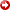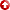--> --> Appendix I
[First Hit]APPENDIX I: THE NARR AND CURRIE (1982) STRESS HISTORY MODEL

Narr and Currie (1982) examined the state of stress within a unit cube of rock subjected to normal horizontal stresses Sx and Sy, and vertical stress Sz:

 Îx = 1 [Sx-v(Sy + Sz)] (1) E

 Î y = 1 [Sy-v(Sz + Sx)] (2) E

 Î z = 1 [Sz-v(Sx + Sy)] (3) E

where E is Young’s Modulus, and n is Poisson’s ratio. E and n are physical properties that are used to describe the rock’s response to stress.

The vertical stress, Sz, is a function of the overburden weight:

 SZ = r(average)gz (4)

The thermal elastic effect due to variation of temperature with depth is included in the Narr and Curries (1982) model by considering temperature range DT experienced by the rock unit during burial and uplift,; and its linear coefficient of thermal expansion a. When these effects are combined with those caused by increasing overburden pressure,equations1, 2, and 3 can be expanded and rewritten:

 EÎx = Sx - v[Sy - Sz] + aE(DT) (5) EÎy = Sy - v[Sz - Sx] + aE(DT) (7) EÎz = Sz - v[Sx - Sy] + aE(DT) (6)

In order to trace the stress history of the unit cube of sediment through a cycle of burial and lithification followed by uplift and erosional unloading, Narr and Currie (1982) determined the magnitude of Sx and Sy inequations5 and 6 as functions of the changes in Sz, ex, and T:

 Sx = v Sz - aE( DT) + EÎx + vEÎy (8) 1-v 1-v 1-v² 1-v²

 Sy = v Sz - aE( DT) + EÎy + vEÎx (9) 1-v 1-v 1-v² 1-v²

The model also takes into account pore fluid pressure. Fracture formation depends upon effective stress, s, which is the difference between the total stress, S, and the pore fluid pressure, P (Hubbert and Ruby, 1959):

 s = S - P (10)Solvingfor S in equation 10 and substituting inequations4, 8, and 9, we arrive at the three components (vertical, horizontal maximum, horizontal minimum) of effective stress in the subsurface:

 sz = r(average)gz - P (11)

 sx = v Sz - aE( DT) + EÎx + vEÎy - P (12) 1-v 1-v 1-v² 1-v²

 sy = v Sz - aE( DT) + EÎy + vEÎx - P (13) 1-v 1-v 1-v² 1-v²Equations11, 12, and 13 express the effective stresses which rocks are subject to during their burial history. Equation 14, the effective minimum horizontal stress, is used in this paper to link with hydrocarbon generation.